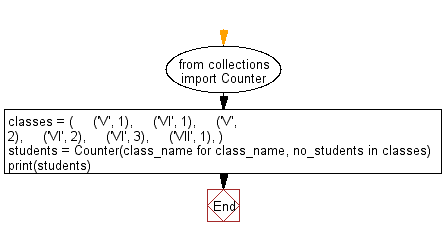﻿ Python: Count the number of students of individual class - w3resource# Python: Count the number of students of individual class

## Python Collections: Exercise-33 with Solution

Write a Python program to count the number of students of individual class.

Sample Solution:

Python Code:

``````from collections import Counter
classes = (
('V', 1),
('VI', 1),
('V', 2),
('VI', 2),
('VI', 3),
('VII', 1),
)
students = Counter(class_name for class_name, no_students in classes)
print(students)
```
```

Sample Output:

```Counter({'VI': 3, 'V': 2, 'VII': 1})
```

Flowchart:## Visualize Python code execution:

The following tool visualize what the computer is doing step-by-step as it executes the said program:

Python Code Editor:

Have another way to solve this solution? Contribute your code (and comments) through Disqus.

What is the difficulty level of this exercise?

Test your Programming skills with w3resource's quiz.

﻿

## Python: Tips of the Day

Use Reversed() In for Loops:

```>>> tasks = ['laundry', 'picking up kids', 'gardening', 'cooking']